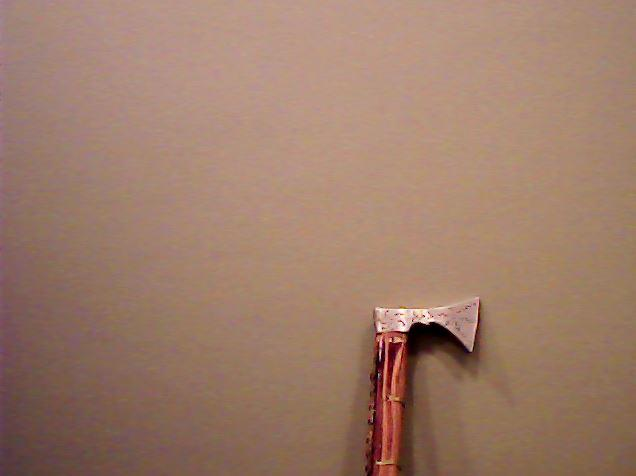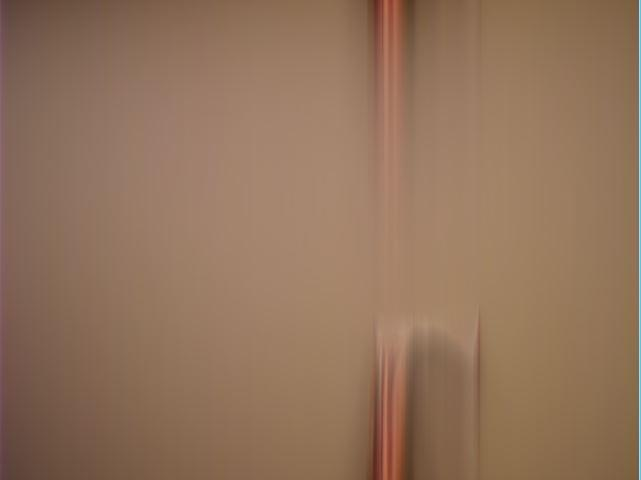Howdy, Stranger!

We are about to switch to a new forum software. Until then we have removed the registration on this forum.

Strange things happen while trying to draw an average frame using a recursive formula.

edited November 2017

Hey ! I want to draw on a screen, an average frame using formula like this: aFrame = 0.99aFrame + 0.01CurrentFrame; (CurrentFrame is an actual frame taken from cam). Ofcourse at the beginning "aFrame" contain diffrent value but with each iteration (new frame loaded) it its closer to CurrentFrame value. After about 10-30 (considering we are using 30 fps camera and the image is static) aFrame should be equal to CurrentFrame and we should get picture like this:Instead, what I get is this:Here is a simple code. What is wrong with these calculations ? Why aren't the rc,gc,bc values getting closer to current frame values ?

import processing.video.*;
import processing.serial.*;

Capture video;
Serial myPort;

void setup()
{
size(640, 480);
video = new Capture(this, width, height, 30);
video.start();
}

void captureEvent(Capture video)
{
}

void draw()
{
image(video, 0, 0);

float rc = 0; // Initialaizing values are 0, so at the start, strange thinks may display
float gc = 0;
float bc = 0;

for (int x = 0; x < video.width; x++ )
{
for (int y = 0; y < video.height; y++ )
{
int loc = x + y * video.width;

color currentColor = video.pixels[loc]; //current values, taken from cam
float r3 = red(currentColor);
float g3 = green(currentColor);
float b3 = blue(currentColor);

rc = rc*0.99 + r3*0.01;        //with each iterations, rc,gc,bc values are closer to current frame
gc = gc*0.99 + g3*0.01;        //so after some time, these values will be almost equal to current r3, g3, b3
bc = bc*0.99 + b3*0.01;

pixels[loc] = color(rc,gc,bc); //I am drawing the values on screen, after some time, I should get clear a clear picture.
}
}
updatePixels();
}
Tagged:

• edited November 2017 Answer ✓

You are treating rc / gc / bc as if they hold the previous value for each pixel -- that is, you are acting as if they are a PGraphics or PImage frame buffer, storing the previous frame (actually, storing several previous frames).

But they aren't. They are only three stored numbers, not 640x480x3 numbers. Because you don't clear them, every value smears into its neighboring values since you only have 3 stored numbers and you never reset them. If you loop (for x (for y ( ) ) then you get vertical smearing, because those pixels are next to each other. If you loop (for y ( for x ( ) ) then you get horizontal smearing.

• edited November 2017 Answer ✓

I think you were trying to do something like this:

import processing.video.*;
Capture video;
PImage last;
color currentColor;
color lastColor;

void setup() {
size(640, 480);
video = new Capture(this, width, height, 30);
last = createImage(width, height, RGB);
video.start();
}

void captureEvent(Capture video) {
}

void draw() {
image(last, 0, 0);
for (int y = 0; y < video.height; y++ ) {
for (int x = 0; x < video.width; x++ ) {
int loc = x + y * video.width;
currentColor = video.pixels[loc]; //current values, taken from cam
lastColor = last.pixels[loc];
last.pixels[loc] = lerpColor(lastColor, currentColor, 0.02);
}
}
last.updatePixels();
}

However note that it is not true that repeatedly blending at a low mix rate will eventually replace image A with image B. Due to float precision (and Zeno's paradox) there are many conditions where you will have afterimage that never clears if you use this approach.

There are several fixes, but you could special-case that: if the distance between currentColor and lastColor is < x, simply replace with currentColor rather than a mix.

• recursive formula

Where?

• @jeremydouglass Thx alot ! @koogs I maybe used a bad word to describe this. I meant a formula in which to calculate value x we are using its previous sample. I know its diffrent than a function thats call itself.